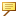Example: Sorting Relations
Example 1: Sorting Regular Relations
For example, you entered the following relations:
d0 = d1 + 3*d2
d2 = d3 + d4
When you sort the relations, the system reorders them based on the order in which they will be evaluated. The sorted relations appear as follows.
d2 = d3 + d4
d0 = d1 + 3*d2
Example 2: Sorting Simultaneous Equations
A set of simultaneous equations is treated as if it is a single relation, and is moved around as a unit. This unit is considered to be dependent upon the union of all the quantities that its individual lines depend upon. A simultaneous equation may contain additional relations that are not being solved, as shown in the following example:
SOLVE
d1 + d2 = 500
d55 = d56 + 50
d56 = d54
d1—d2 = 0
FOR d1 d2
The extra lines are sorted within the body of the simultaneous equation, producing the following result:
SOLVE
d1 + d2 = 500
d1-d2 = 0
d56 = d54
d55 = d56 + 50
FOR d1 d2Lines are never inserted into or removed from simultaneous equations.